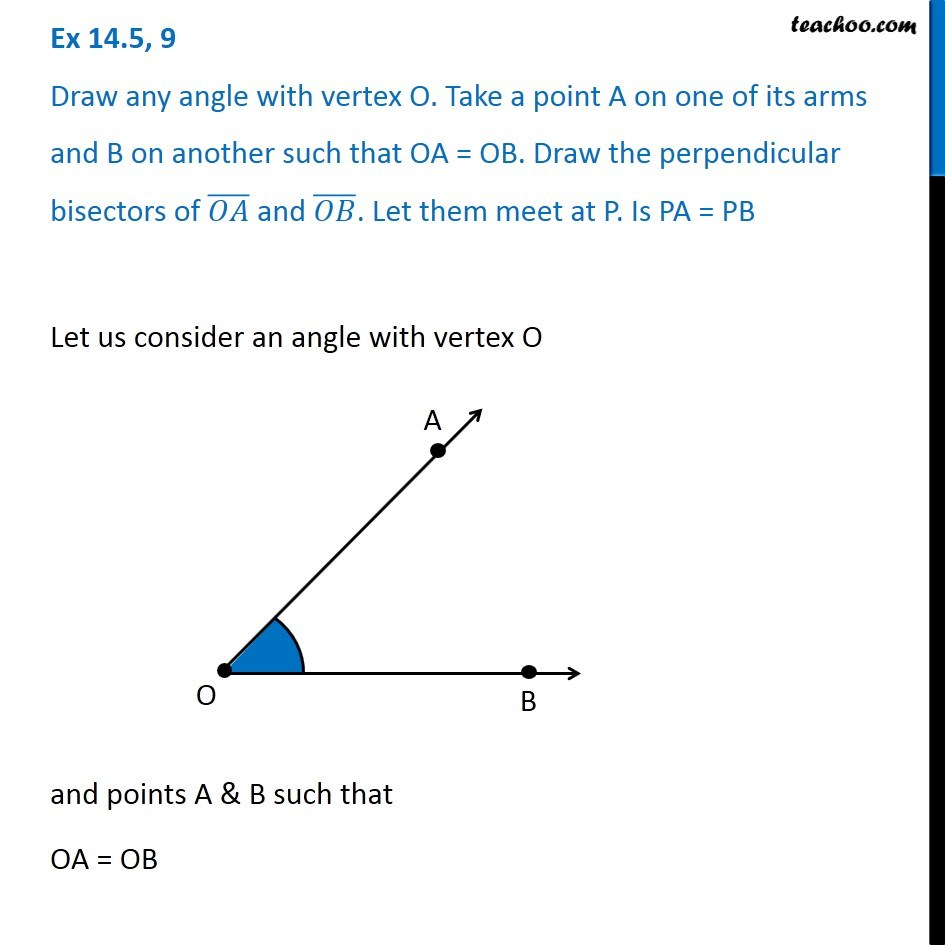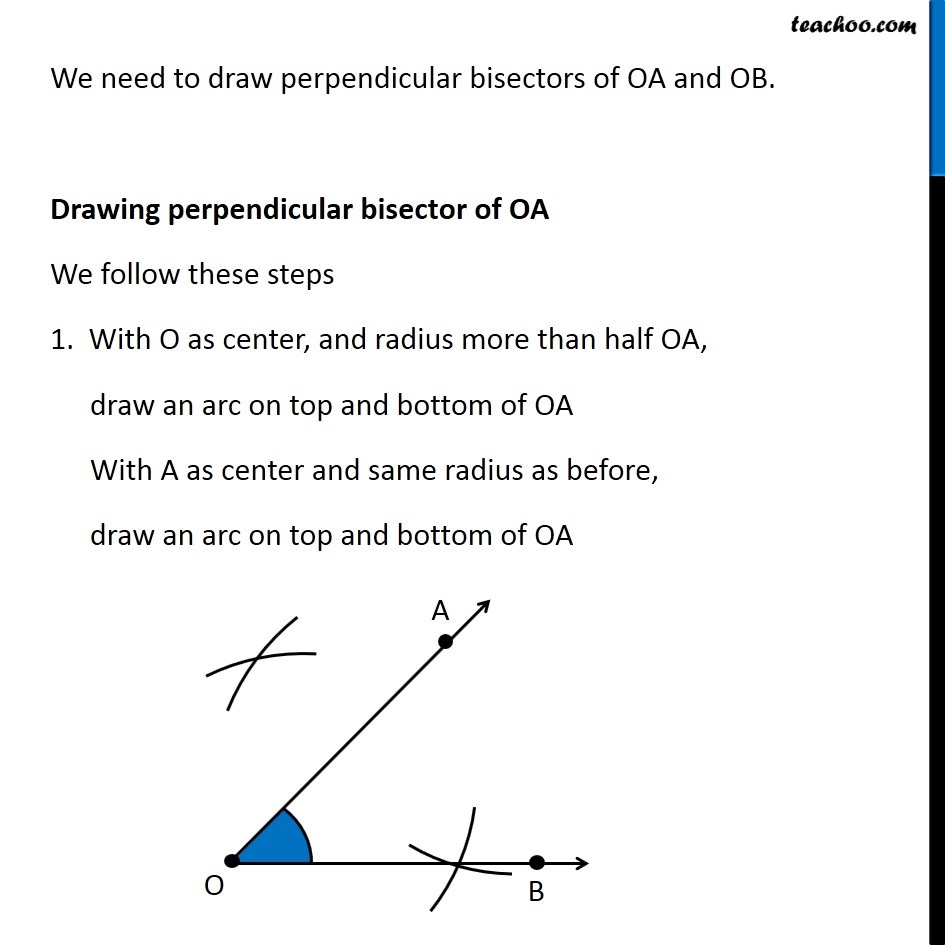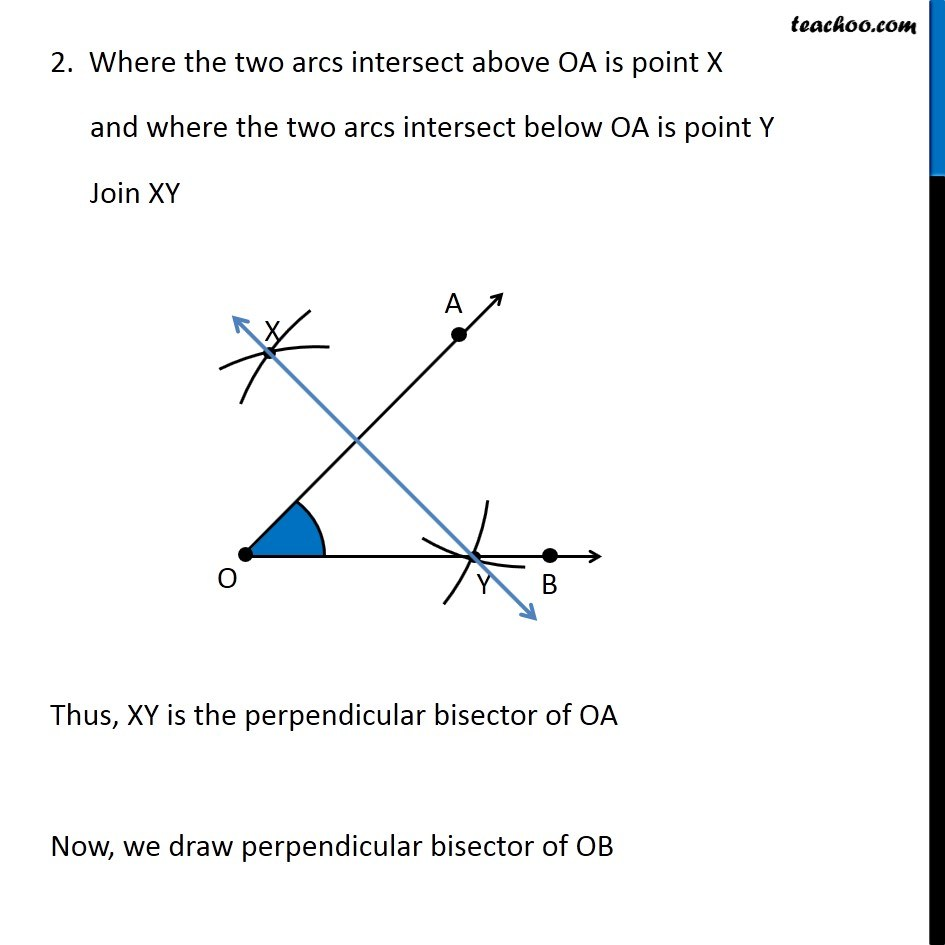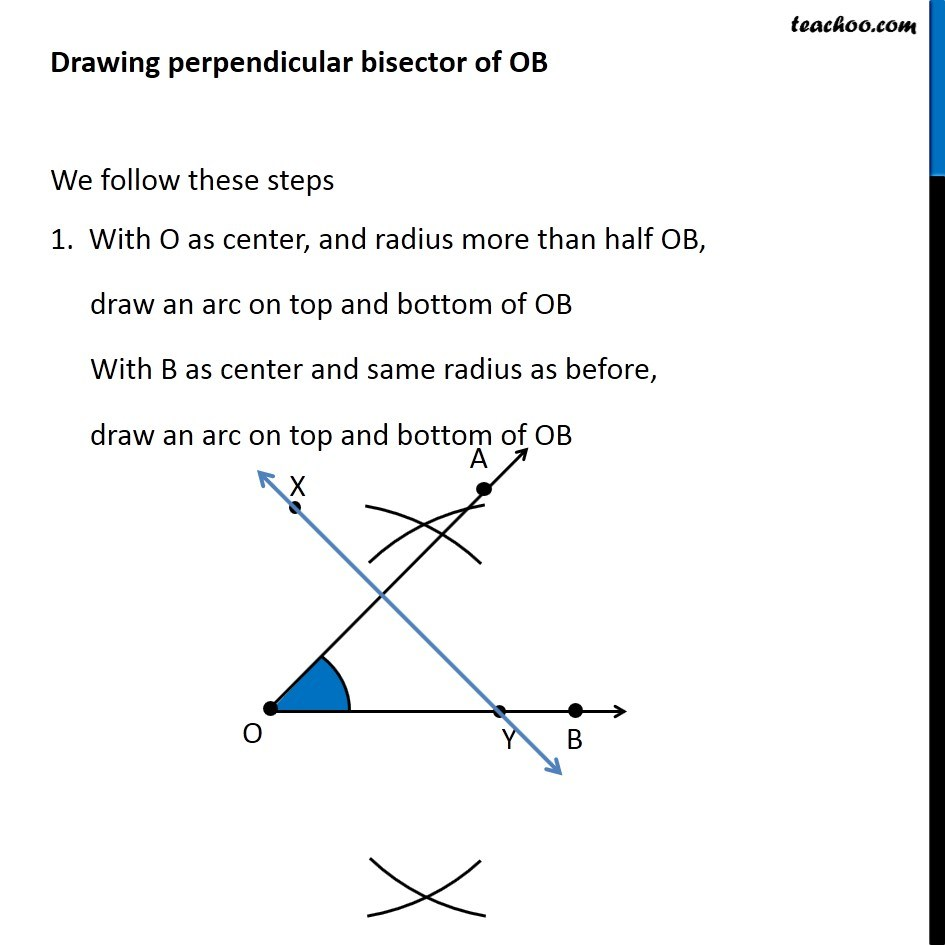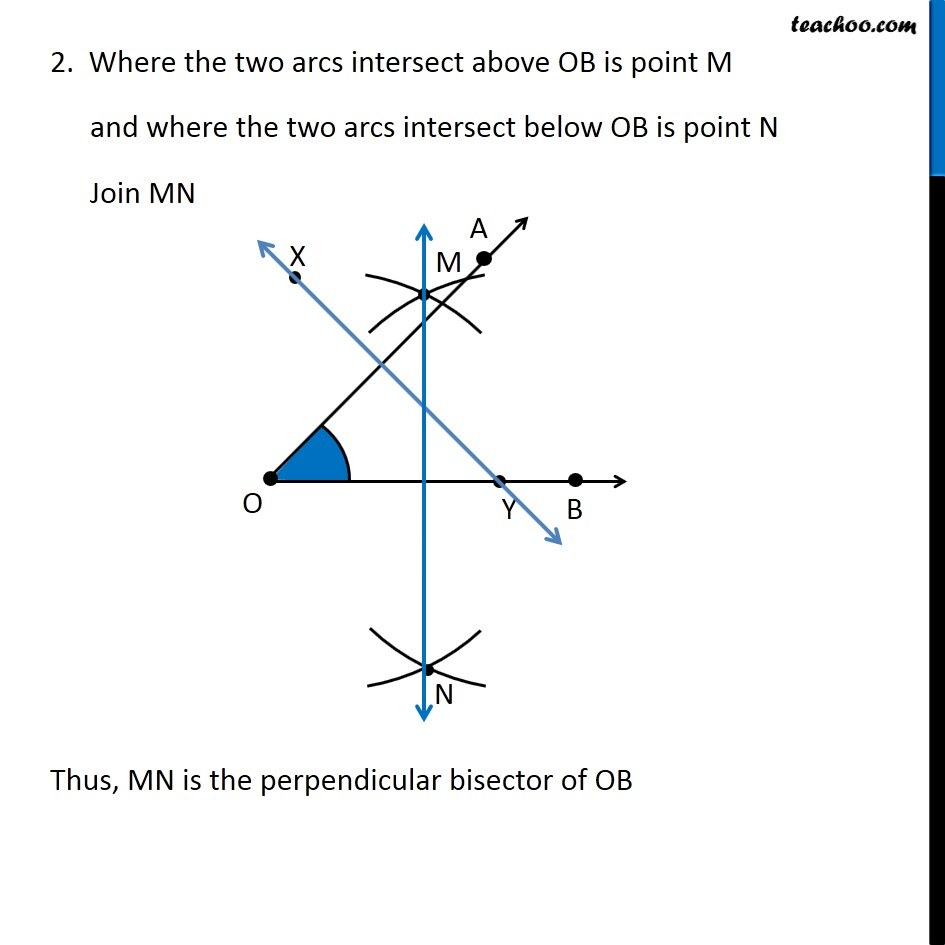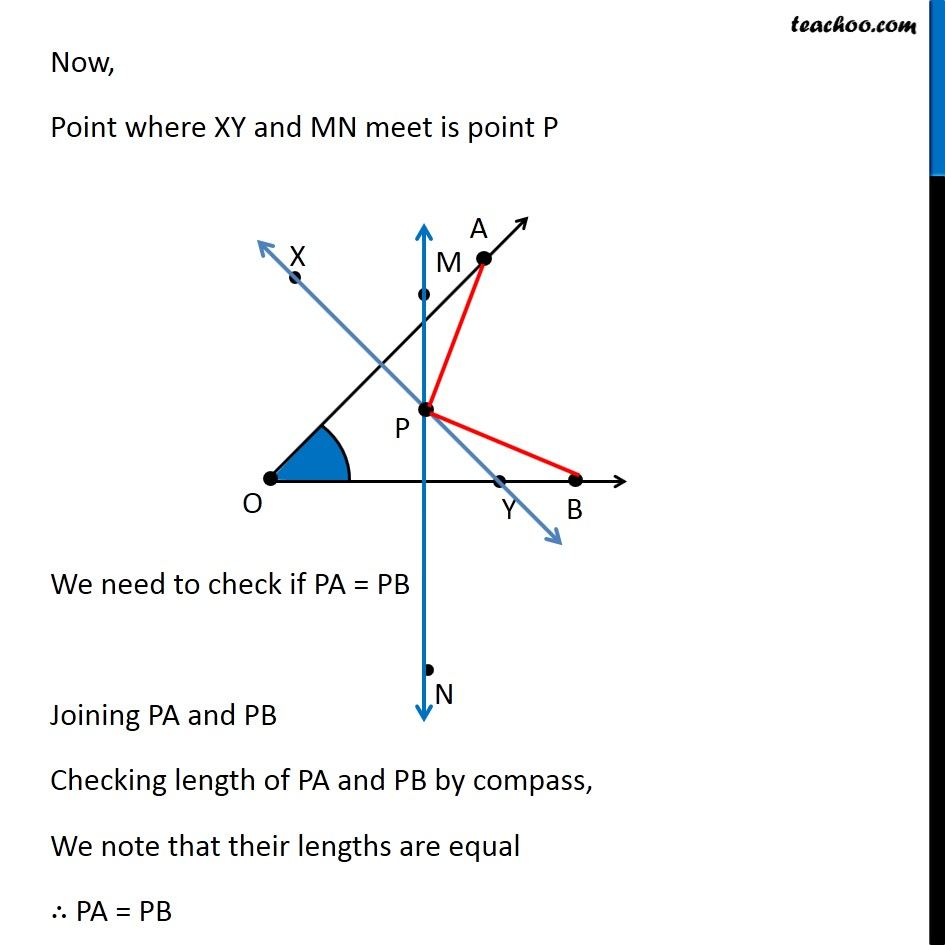1. Chapter 14 Class 6 Practical Geometry
2. Serial order wise
3. Ex 14.5

Transcript

Ex 14.5, 9 Draw any angle with vertex O. Take a point A on one of its arms and B on another such that OA = OB. Draw the perpendicular bisectors of (𝑂𝐴) ̅ and (𝑂𝐵) ̅. Let them meet at P. Is PA = PB Let us consider an angle with vertex O and points A & B such that OA = OB We need to draw perpendicular bisectors of OA and OB. Drawing perpendicular bisector of OA We follow these steps 1. With O as center, and radius more than half OA, draw an arc on top and bottom of OA With A as center and same radius as before, draw an arc on top and bottom of OA 2. Where the two arcs intersect above OA is point X and where the two arcs intersect below OA is point Y Join XY Thus, XY is the perpendicular bisector of OA Now, we draw perpendicular bisector of OB Drawing perpendicular bisector of OB We follow these steps 1. With O as center, and radius more than half OB, draw an arc on top and bottom of OB With B as center and same radius as before, draw an arc on top and bottom of OB 2. Where the two arcs intersect above OB is point M and where the two arcs intersect below OB is point N Join MN Thus, MN is the perpendicular bisector of OB Now, Point where XY and MN meet is point P We need to check if PA = PB Joining PA and PB Checking length of PA and PB by compass, We note that their lengths are equal ∴ PA = PB

Ex 14.5

Chapter 14 Class 6 Practical Geometry
Serial order wise